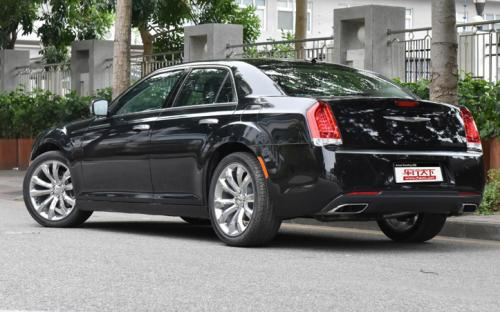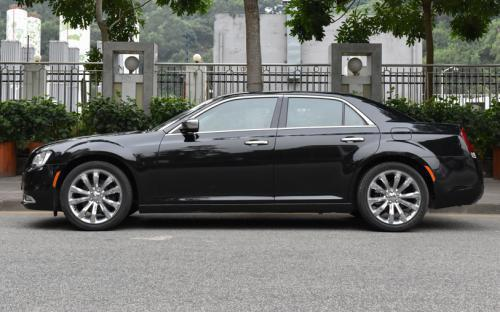### 克莱斯勒(进口) 克莱斯勒300C(进口)9 种颜色可选2016款最低售价：39.99 万元起

5066(mm)1902(mm)1488(mm)##### 配置亮点：
• 胎压监测装置

• ISOFIX儿童座椅接口

• 车身稳定控制(ESC/ESP/DSC等)

• 电动天窗

• 定速巡航

• 后倒车雷达

• 真皮座椅

• GPS导航系统

• 氙气大灯

• 后视镜加热

• 提交
2016款 3.0L 超越版 (128张)
• 2016款 3.0L 超越版 (128张)
• 2016款 3.6L 尊崇版 (97张)
• 克莱斯勒(进口) 克莱斯勒300C(进口) 绕车实拍• 克莱斯勒(进口) 克莱斯勒300C(进口) 在售车型

排量 车型 厂商指导价 本地最低报价 购车工具
3.0L
3.0L 超越版 8挡手自一体
39.99万
3.6L 尊崇版 8挡手自一体
43.19万
3.6L
3.0L 超越版 8挡手自一体
39.99万
3.6L 尊崇版 8挡手自一体
43.19万

克莱斯勒(进口) 克莱斯勒300C(进口) 经销商

查看更多 >>

### 克莱斯勒(进口) 克莱斯勒300C(进口) 动力加速

克莱斯勒300C(进口) 0-100公里加速时间分布在 0.0-秒 属于 超跑级

动力级别 加速时间 车型

克莱斯勒(进口) 克莱斯勒300C(进口) 视频

克莱斯勒(进口) 克莱斯勒300C(进口) 新闻资讯

# 克莱斯勒新款300C正式上市 售价39.99万起

上市新车 超过8433次关注

2015年12月2日，克莱斯勒宣布新款300C正式上市，新车克莱斯勒新款300C针对一些细节进行了改进，水晶的网状进气格栅，而前保险杠、前大灯、雾灯造型也有所变化；提供...

# 非主流之选 50万内进口中型车推荐

导购 超过2363次关注

谈到克莱斯勒300C，我相信很多国内消费者都不会感到陌生。当年第十代车型国产后，凭借豪华且大气的外观曾经得到不少国内消费者的青睐，不过受到油耗过高以及后来厂...

# 克莱斯勒300C 3.0L性能测试

评测 超过2537次关注

这台克莱斯勒300C3.0L卓越版（以下简称300C）配备了一台3.0LPentastarVVTV6自然吸气发动机，最大功率234马力/6350rpm，峰值扭矩285牛·米/4400rpm，指导售价在40万...

# 克莱斯勒300S锋尚版实拍 加入运动元素

导购 超过3581次关注

谈及克莱斯勒300C，相信许多国内消费者和车友都不陌生，其凭借着豪华大气的外观赢得了不少喜欢美式豪放风格车迷的喜爱。前段时间，厂家为了进一步丰富300C的产品线...

# 克莱斯勒300C 3.0L于2014北京车展上市

新闻 超过2307次关注

在本届北京车展上，克莱斯勒300C3.0L车型正式上市，该车仅有一款，售价为39.99万元。

# 克莱斯勒300C 3.0L车型首发 预售40万元

新闻 超过2435次关注

克莱斯勒正式发布了300C3.0L车型，相比于在售的3.6L车型，克莱斯勒300C3.0L车型将会有更高的性价比。根据官方目前透露的消息，该车型将于今年4月的北京车展后正式上...

# 克莱斯勒召回进口300S车型 涉及151辆

新闻 超过2443次关注

日前，克莱斯勒（中国）汽车销售有限公司向国家质检总局备案了召回计划，将自2014年5月15日起，召回部分进口款克莱斯勒2013款/2014款300S车型。据该公司统计，在中...

猜你喜欢

﻿
• 快速找车
• 选择品牌
• 选择品牌
• A  奥迪
• A  阿斯顿·马丁
• A  阿尔法·罗密欧
• B  宝沃
• B  布加迪
• B  巴博斯
• B  保时捷
• B  宾利
• B  奔驰
• B  宝马
• B  本田
• B  别克
• B  标致
• B  比亚迪
• B  宝骏
• B  北汽制造
• B  北汽新能源
• B  北汽幻速
• B  北汽威旺
• B  北京汽车
• B  奔腾
• B  北汽绅宝
• C  长安
• C  长安商用
• C  长城
• C  昌河
• D  大众
• D  道奇
• D  DS
• D  东南
• D  东风风神
• D  东风风行
• D  东风小康
• D  东风风度
• D  东风
• F  福特
• F  丰田
• F  菲亚特
• F  法拉利
• F  福田
• F  福迪
• F  福汽启腾
• G  观致
• G  广汽传祺
• G  广汽吉奥
• G  GMC
• H  红旗
• H  汉腾汽车
• H  哈弗
• H  哈飞
• H  海格
• H  海马
• H  华颂
• H  黄海
• H  华泰
• H  恒天
• J  吉利汽车
• J  捷豹
• J  Jeep
• J  江淮
• J  江铃
• J  金杯
• J  九龙
• J  金旅
• K  凯翼
• K  凯迪拉克
• K  克莱斯勒
• K  科尼塞克
• K  卡威
• K  开瑞
• L  路虎
• L  林肯
• L  劳斯莱斯
• L  兰博基尼
• L  雷克萨斯
• L  铃木
• L  雷诺
• L  理念
• L  力帆
• L  莲花汽车
• L  猎豹
• L  路特斯
• L  陆风
• M  马自达
• M  MG
• M  MINI
• M  玛莎拉蒂
• M  摩根
• M  迈凯轮
• N  纳智捷
• O  欧宝
• O  讴歌
• O  欧朗
• Q  奇瑞
• Q  起亚
• Q  启辰
• R  日产
• R  荣威
• R  瑞麒
• S  三菱
• S  斯威汽车
• S  萨博
• S  smart
• S  斯柯达
• S  斯巴鲁
• S  思铭
• S  双龙
• S  上汽大通
• S  双环
• T  特斯拉
• T  腾势
• W  沃尔沃
• W  五菱汽车
• W  五十铃
• W  威兹曼
• W  威麟
• X  现代
• X  雪佛兰
• X  雪铁龙
• X  西雅特
• Y  一汽
• Y  英菲尼迪
• Y  英致
• Y  依维柯
• Y  野马汽车
• Y  永源
• Z  众泰
• Z  中华
• Z  中兴
• Z  知豆
• 选择车系
• 选择车系
• 车型对比
• 选择品牌
• 选择品牌
• A  奥迪
• A  阿斯顿·马丁
• A  阿尔法·罗密欧
• B  宝沃
• B  布加迪
• B  巴博斯
• B  保时捷
• B  宾利
• B  奔驰
• B  宝马
• B  本田
• B  别克
• B  标致
• B  比亚迪
• B  宝骏
• B  北汽制造
• B  北汽新能源
• B  北汽幻速
• B  北汽威旺
• B  北京汽车
• B  奔腾
• B  北汽绅宝
• C  长安
• C  长安商用
• C  长城
• C  昌河
• D  大众
• D  道奇
• D  DS
• D  东南
• D  东风风神
• D  东风风行
• D  东风小康
• D  东风风度
• D  东风
• F  福特
• F  丰田
• F  菲亚特
• F  法拉利
• F  福田
• F  福迪
• F  福汽启腾
• G  观致
• G  广汽传祺
• G  广汽吉奥
• G  GMC
• H  红旗
• H  汉腾汽车
• H  哈弗
• H  哈飞
• H  海格
• H  海马
• H  华颂
• H  黄海
• H  华泰
• H  恒天
• J  吉利汽车
• J  捷豹
• J  Jeep
• J  江淮
• J  江铃
• J  金杯
• J  九龙
• J  金旅
• K  凯翼
• K  凯迪拉克
• K  克莱斯勒
• K  科尼塞克
• K  卡威
• K  开瑞
• L  路虎
• L  林肯
• L  劳斯莱斯
• L  兰博基尼
• L  雷克萨斯
• L  铃木
• L  雷诺
• L  理念
• L  力帆
• L  莲花汽车
• L  猎豹
• L  路特斯
• L  陆风
• M  马自达
• M  MG
• M  MINI
• M  玛莎拉蒂
• M  摩根
• M  迈凯轮
• N  纳智捷
• O  欧宝
• O  讴歌
• O  欧朗
• Q  奇瑞
• Q  起亚
• Q  启辰
• R  日产
• R  荣威
• R  瑞麒
• S  三菱
• S  斯威汽车
• S  萨博
• S  smart
• S  斯柯达
• S  斯巴鲁
• S  思铭
• S  双龙
• S  上汽大通
• S  双环
• T  特斯拉
• T  腾势
• W  沃尔沃
• W  五菱汽车
• W  五十铃
• W  威兹曼
• W  威麟
• X  现代
• X  雪佛兰
• X  雪铁龙
• X  西雅特
• Y  一汽
• Y  英菲尼迪
• Y  英致
• Y  依维柯
• Y  野马汽车
• Y  永源
• Z  众泰
• Z  中华
• Z  中兴
• Z  知豆
• 选择车系
• 选择车系
• 选择车型
• 选择车型
• 意见反馈